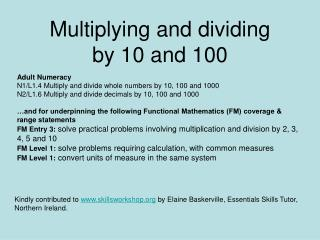DownloadDownload PresentationMultiplying and dividing by 10 and 100

# Multiplying and dividing by 10 and 100

Télécharger la présentation## Multiplying and dividing by 10 and 100

- - - - - - - - - - - - - - - - - - - - - - - - - - - E N D - - - - - - - - - - - - - - - - - - - - - - - - - - -
##### Presentation Transcript

1. Multiplying and dividing by 10 and 100 Adult Numeracy N1/L1.4 Multiply and divide whole numbers by 10, 100 and 1000 N2/L1.6 Multiply and divide decimals by 10, 100 and 1000 …and for underpinning the following Functional Mathematics (FM) coverage & range statements FM Entry 3:solve practical problems involving multiplication and division by 2, 3, 4, 5 and 10 FM Level 1: solve problems requiring calculation, with common measures FM Level 1: convert units of measure in the same system Kindly contributed to www.skillsworkshop.org by Elaine Baskerville, Essentials Skills Tutor, Northern Ireland.

2. Multiply by 10 – move the digits one place to the left 5 4 3 7 1 8 0 5 Multiply by 100 – move the digits two places to the left 0 7 6 0 2 0 0 8 3

3. Divide by 10 – move the digits one place to the right 0 5 4 0 3 7 1 8 5 0 Divide by 100 – move the digits two places to the right 0 0 7 2 0 0 8 9 5